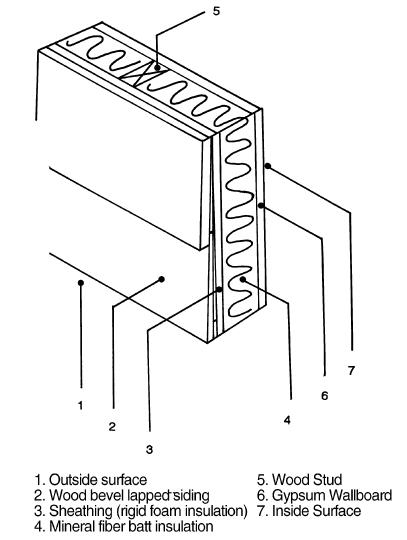﻿﻿ Heat Loss Calculations | NaturalGasEfficiency.org

# Heat Loss Calculations

#### Introduction

The formulas for calculating heat loss and heat gain are not complex. The complexity comes from the large number of assumptions that must be made in order to come up with the values that are input into the simple formulas. Very basic calculations are used to estimate the annual heating (and cooling) costs for a space using only a single formula for each major heat loss (gain) area. More complex methods use a computer to repeat the same simple formula 8,760 times, once for each hour of the year, using hourly variable assumptions. Complex models consider wind speed and exposure, solar isolation and cloud cover, occupancy rates, and other factors that may impact annual energy usage.

The accuracy of the results will be determined by the assumptions made for input into the formulas. Running a complex 8,760 computer model will not produce better results if the assumptions entered are way out of line with real world conditions. (Garbage in garbage out.)

This section deals mainly with conduction and infiltration heat loss; for information on heat gain and solar heat gain, see Heat Gain.

### The Basic Formulas

The basic formula for conductive heat transfer is:

(Area) x (U-Value) x (Temperature Difference) = BTUs/Hour

(Area) x (U-Value) x (Seasonal Degree Days x 24 Hours) = BTUs/Season

Area = Square Feet
U-Value = Transmission Factor = 1/R-Value = inverse of the R-value
Temperature Difference = Delta-T = ((Inside Temperature) – (Outside Temperature))
The basic formula for infiltration and heat transfer by moving air is:

(Volume) x (Rate of Change) x (Specific Heat of Air) x (Temp Diff) = BTUs/Hour

(Volume) x (Rate of Change) x (Specific Heat of Air) x (DD x 24 Hours) = BTUs/Season

Volume = cubic feet
Rate of Change = Air Changes per Hour; fan CFH, etc
Specific Heat of Air = BTUs per cubic foot of air = 0.018 typical
Temp Diff = Difference in temperature between the air being exchanged

Area and Volume
Area is always entered as square footage and volume is cubic foot. Basic math skills are required to calculate each area of different construction, materials and R-Value. All areas that form a barrier between indoor heated space and outdoor space must be determined.
Estimating the air change rate is probably the largest single variable in heat loss/gain calculations. General Rules of Thumb vary under many conditions. A good starting point is:
Residential New/Tight Construction = 0.25 – 0.5 air change per hour

Residential older homes = 0.5 – 1.0 air change per hour

Residential old, un-insulated houses with single pane windows 1.0 – 1.5 air change per hour

Commercial Spaces with limited exterior openings and no power ventilation = 0.5 ac/hour

Commercial Spaces with lots of door openings/people traffic = 1.0 – 2.0 ac/hour

Industrial Spaces with Over-head doors, windows, power ventilation = 0.5 – 3.0 ac/hour (the larger the space the LOWER the ac/hour)
Blower Doors can precisely measure the air infiltration rate of residential and small commercial buildings. For more information see Blower Doors.
R-Values and U-Values
R-Values are the common rating used in materials, however, it is the U-Value that is used in the formulas. A U-Value is the inverse of an R-Value (ie: R-2 = U-1/2).

R-Values can be added; U-Values can not. Therefore, the Total R-Value must be determined by adding up all the individual R-Values of a composite material, and then convert it to a U-Value to enter into the formula. (NOTE: computer programs may allow direct input in R-Values and then do the conversion in the program.)Example: Residential Wall Assembly R-Value showing additive method

 Component R-value Wall – Outside Air Film 0.17 Siding – Wood Bevel 0.80 Plywood Sheathing – 1/2″ 0.63 3 1/2″ Fiberglass Batt 11.00 1/2″ Drywall 0.45 Inside Air Film 0.68 Total Wall Assembly R-Value 13.73

Total R-Value = R-13.73

U-Value = 1/13.73 = 0.0728

Note that the wall section is not 100% homogeneous; at least 20% of the typical residential wall is framing materials. Therefore, to be more precise, 80% of the wall is R-13.73 and 20% of the wall is R-4.248 (replacing R-11 insulation with R-3 wood framing). Often the ‘Framing Factor’ is not considered in residential calculations.

There are many sources of R-Value ratings, including manufacturers, ASHRAE, and ACCA.

### Examples

What is the hourly heat loss of 500 square foot of wall area of R-13 construction when there is a 70F Delta-T?

500 x 1/13 x 70 = 2,692 BTUs per Hour

How many BTUs can be saved by adding R-10 to the roof of a 10,000 square foot warehouse that has an existing R-5 roof located in a 6,000 Degree Day climate?

10,000 x 1/5 x 6,000 x 24 = 288,000,000 BTUs per Season

10,000 x 1/15 x 6,000 x 24 = 96,000,000 BTUs per Season

288 – 96 = 192 Million BTUs per Season Saved

Using a little Algebra, we could also calculate it with one formula:

10,000 x (1/5 – 1/15) x 6,000 x 24 = 192,000,000 BTUs per Season

How many BTU’s per hour can be saved by reducing ventilation air from 1,000 CFM to 750 CFM when there is a 70F Delta-T?

(1,000 – 750 CFM) x 60 Min/Hour x 0.018 x 70 = 18,900 BTUs per Hour

The ACCA is the publisher of Manual J (Residential Load Calculations) and Manual N (Small Commercial Load Calculations) the long-recognized leader in load estimation methods. For information about these and other resources, visit the ACCA’s web site at:

Air Conditioning Contractors of America
Arlington, VA 22206
Telephone: (703) 575-4477

www.acca.org

The American Society of Heating Refrigeration and Air Conditioning Engineers, ASHRAE publishes one of four Handbooks every year. Included in the Fundamentals edition is a complete energy calculations section that list several pages of R-Values of construction materials.

ASHRAE
1791 Tullie Circle, N.E.
Atlanta, GA 30329
Telephone: (800) 527-4723, (404) 636-8400

www.ashrae.org